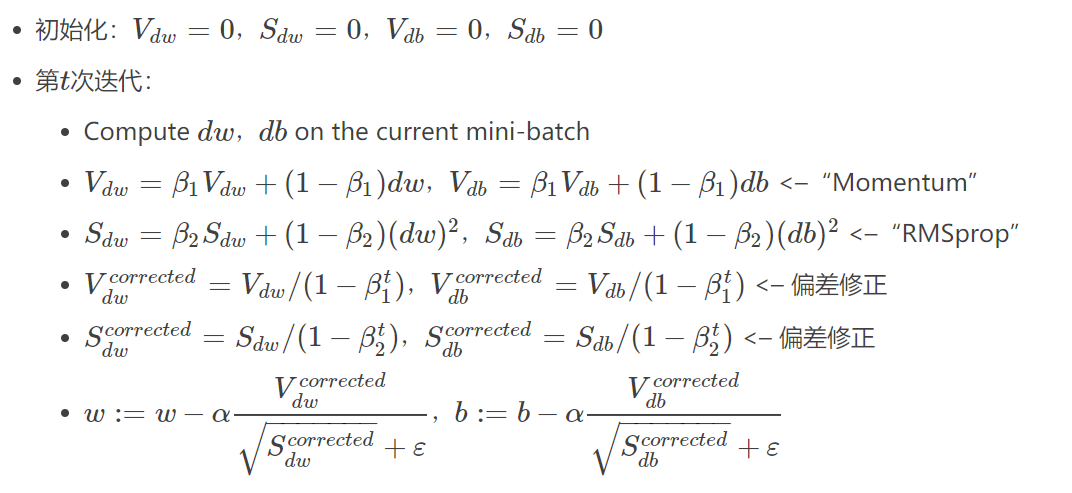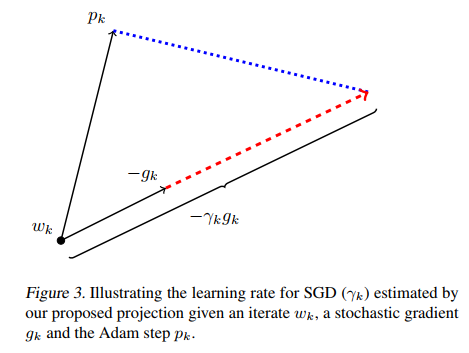laffitto

• 累计撰写 88 篇文章
• 累计创建 34 个标签
• 累计收到 101 条评论

### 目 录CONTENT# 深度学习优化算法总结2020-08-22 / 0 评论 / 0 点赞 / 265 阅读 / 6,450 字

## 优化算法总体框架

1. 计算目标函数关于当前参数的梯度：$g_{t}=\nabla f\left(w_{t}\right)$
2. 根据历史梯度计算一阶动量和二阶动量：$m_{t}=\phi\left(g_{1}, g_{2}, \cdots, g_{t}\right) ; V_{t}=\psi\left(g_{1}, g_{2}, \cdots, g_{t}\right)$
3. 计算当前时刻的下降梯度：$\eta_{t}=\alpha \cdot m_{t} / \sqrt{V_{t}}$
4. 根据下降梯度进行更新： $w_{t+1}=w_{t}-\eta_{t}$

### SGDM（SGD with Momentum）

$m_{t}=\beta_{1} \cdot m_{t-1}+\left(1-\beta_{1}\right) \cdot g_{t}$

### SGD with Nesterov Acceleration

$g_{t}=\nabla f\left(w_{t}-\alpha \cdot m_{t-1} / \sqrt{V_{t-1}}\right)$

$V_{t}=\sum_{\tau=1}^{t} g_{\tau}^{2}$

$V_{t}=\beta_{2} * V_{t-1}+\left(1-\beta_{2}\right) g_{t}^{2}$

SGD的一阶动量：

$m_{t}=\beta_{1} \cdot m_{t-1}+\left(1-\beta_{1}\right) \cdot g_{t}$

$V_{t}=\beta_{2} * V_{t-1}+\left(1-\beta_{2}\right) g_{t}^{2}$$g_{t}=\nabla f\left(w_{t}-\alpha \cdot m_{t-1} / \sqrt{V_{t}}\right)$

### 选取方法

• 对于稀疏数据，尽量使用学习率可自适应的优化方法，不用手动调节，而且最好采用默认值

• SGD通常训练时间更长，但是在好的初始化和学习率调度方案的情况下，结果更可靠

• 如果在意更快的收敛，并且需要训练较深较复杂的网络时，推荐使用学习率自适应的优化方法。

### 问题1：可能不收敛

$\eta_{t}=\alpha \cdot m_{t} / \sqrt{V_{t}}$

$V_{t}=\max \left(\beta_{2} * V_{t-1}+\left(1-\beta_{2}\right) g_{t}^{2}, V_{t-1}\right)$

## 一点优化算法的使用技巧

1. 何时切换优化算法
2. 切换算法后的学习率

$\eta_{t}^{A d a m}=\left(\alpha / \sqrt{V_{t}}\right) \cdot m_{t}$

$\eta_{t}^{S G D}=\alpha^{S G D} \cdot g_{t}$

$\eta_^$ 必定可以分解为$\eta_{t}^{A d a m}$所在方向及其正交方向上的两个方向之和，那么其在$\eta_{t}^{A d a m}$ 方向上的投影就意味着SGD在Adam算法决定的下降方向上前进的距离，而在 $\eta_{t}^{A d a m}$ 的正交方向上的投影是 SGD 在自己选择的修正方向上前进的距离。$\operatorname{proj}_{\eta_{t}^{S G D}}=\eta_{t}^{A d a m}$

$\alpha_{t}^{S G D}=\left(\left(\eta_{t}^{\text {Adam}}\right)^{T} \eta_{t}^{\text {Adam}}\right) /\left(\left(\eta_{t}^{\text {Adam}}\right)^{T} g_{t}\right)$

$\lambda_{t}^{S G D}=\beta_{2} \cdot \lambda_{t-1}^{S G D}+\left(1-\beta_{2}\right) \cdot \alpha_{t}^{S G D}$ $\tilde{\lambda}_{t}^{S G D}=\lambda_{t}^{S G D} /\left(1-\beta_{2}^{t}\right)$

. 每次迭代玩都计算一下SGD接班人的相应学习率，如果发现基本稳定了，那就SGD以$\tilde{\lambda}_{t}^{S G D}$ 为学习率接班前进。

G-VKJB8HJHH8